> 文档中心 > 【JavaSE系列】基础经典编程题

# 一、判断是不是素数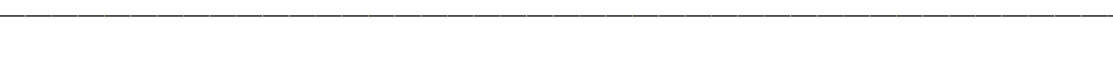``import java.util.Scanner;public class TestDemo_4 {    /**     * 判断是不是素数的方法     * @param num -> num是所要判断的数字     * @return false 说明不是素数；true说明是素数     */    public static boolean isPrime(int num){ for (int i = 2; i < num; i++) {     if (num % i == 0){  return false;     } } return true;  //代码如果可以执行到这一行，说明前面的不满足条件，即到这一行的话 num是素数    }    public static void main(String[] args) { Scanner scanner = new Scanner(System.in); int num = scanner.nextInt(); if (isPrime(num)){     System.out.println(num+"是素数"); }else{     System.out.println(num+"不是素数"); }    }}``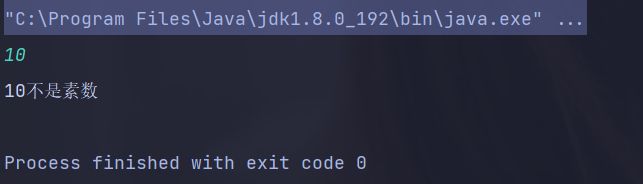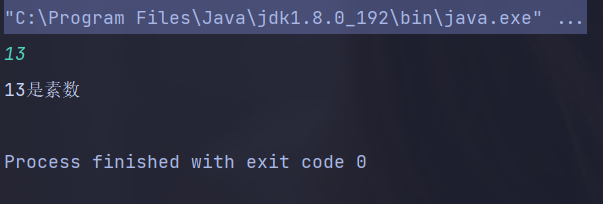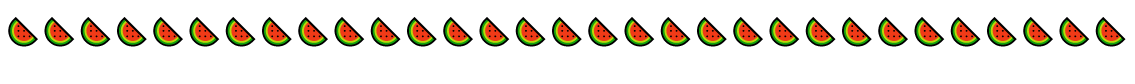``import java.util.Scanner;public class TestDemo_4 {    /**     * 判断是不是素数的方法     * @param num -> num是所要判断的数字     * @return false 说明不是素数；true说明是素数     */    public static boolean isPrime(int num){ for (int i = 2; i <= num/2; i++) {     if (num % i == 0){  return false;     } } return true;  //代码如果可以执行到这一行，说明前面的不满足条件，即到这一行的话 num是素数    }    public static void main(String[] args) { Scanner scanner = new Scanner(System.in); int num = scanner.nextInt(); if (isPrime(num)){     System.out.println(num+"是素数"); }else{     System.out.println(num+"不是素数"); }    }}````import java.util.*;public class TestDemo_4 {    /**     * 判断是不是素数的方法     * @param num -> num是所要判断的数字     * @return false 说明不是素数；true说明是素数     */    public static boolean isPrime(int num){ for (int i = 2; i <= Math.sqrt(num); i++) {     if (num % i == 0){  return false;     } } return true;  //代码如果可以执行到这一行，说明前面的不满足条件，即到这一行的话 num是素数    }    public static void main(String[] args) { Scanner scanner = new Scanner(System.in); int num = scanner.nextInt(); if (isPrime(num)){     System.out.println(num+"是素数"); }else{     System.out.println(num+"不是素数"); }    }}``

输出结果：# 二、打印素数思路：

``import java.util.*;public class TestDemo_4 {    /**     * 判断某个数字是不是素数的方法     * @param num -> num是所要判断的数字     * @return false 说明不是素数；true说明是素数     */    public static boolean isPrime(int num){ for (int i = 2; i <= Math.sqrt(num); i++) {     if (num % i == 0){  return false;     } } return true;  //代码如果可以执行到这一行，说明前面的不满足条件，即到这一行的话 num是素数    }    public static void main(String[] args) { for (int i = 2; i < 100; i++) {   if (isPrime(i)){  System.out.print(i+" ");     } }    }}``

输出结果：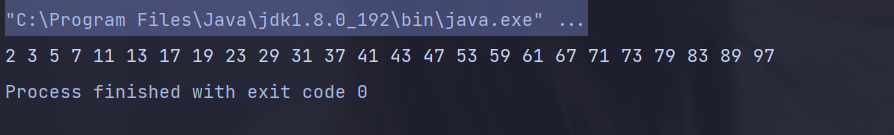# 三、输出闰年``import java.util.*;public class TestDemo_4 {    /**     * 判断传入的数据是不是闰年的方法     * @param year 输入参数是year     * @return 是闰年返回true，不是返回false     */    public static boolean isLeapYear(int year){ if (year % 100 != 0 && year % 4 == 0 || year % 400 ==0){     return true; } return false;    }    public static void main(String[] args) { for (int year = 1000; year <= 2000; year++) {     if (isLeapYear(year)){  System.out.print(year+" ");     } }    }}``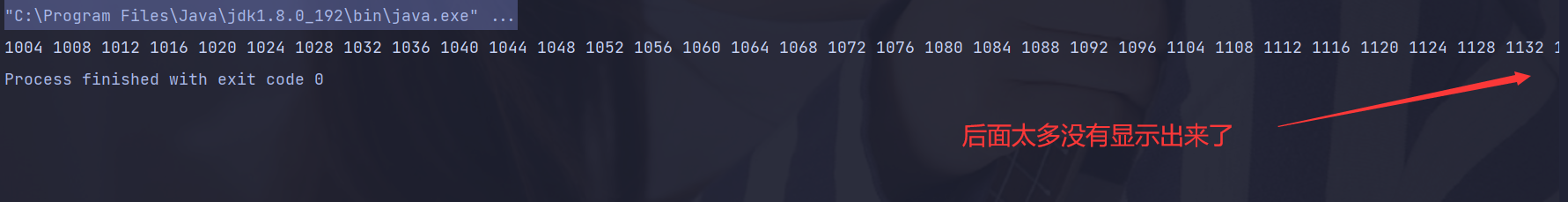# 四、输出乘法口诀表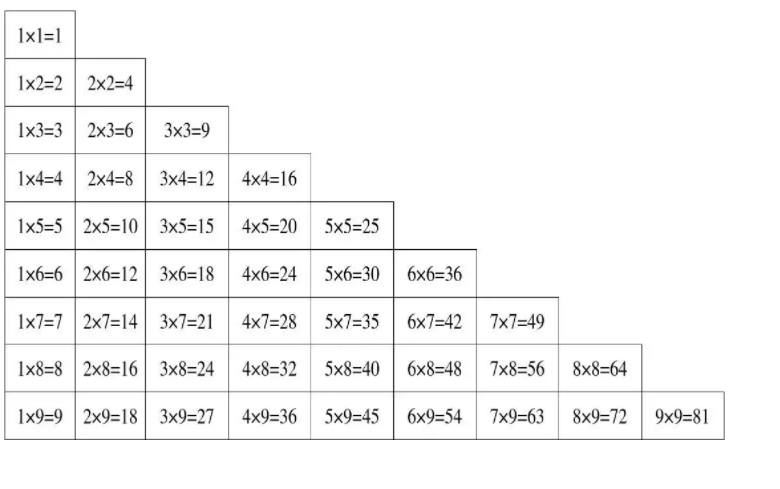代码示例：

``import java.util.*;public class TestDemo_4 {    public static void func(){ for (int i = 1; i <= 9; i++) {     for (int j = 1; j <= i; j++) {  System.out.print(j+"*"+i+"="+i*j+" ");     }     System.out.println(); }    }    public static void main(String[] args) { func();    }}``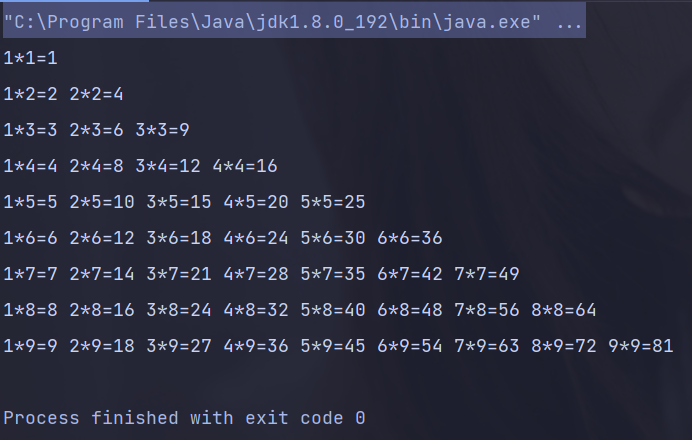# 五、年龄打印

``import java.util.*;public class TestDemo_4 {    public static void main(String[] args) { Scanner scanner = new Scanner(System.in); int age = scanner.nextInt(); if (0<=age && age<=18){     System.out.println("少年"); }else if(19<=age && age<=28){     System.out.println("青年"); }else if (29<=age && age<=55){     System.out.println("中年"); }else{     System.out.println("老年"); }    }}``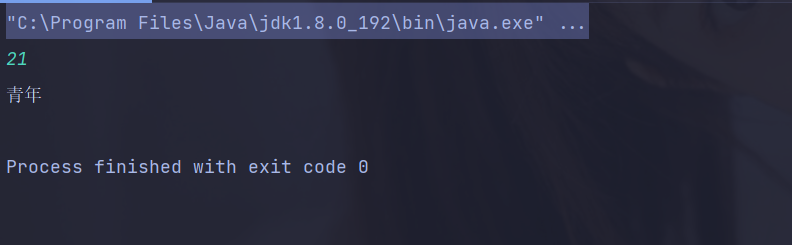最后的最后需要小小说明一下，每一道题目都有若干种解法，上述只是其中一部分解法，如果大家感兴趣的话，还可以尝试其他的解法哦！！！！！！